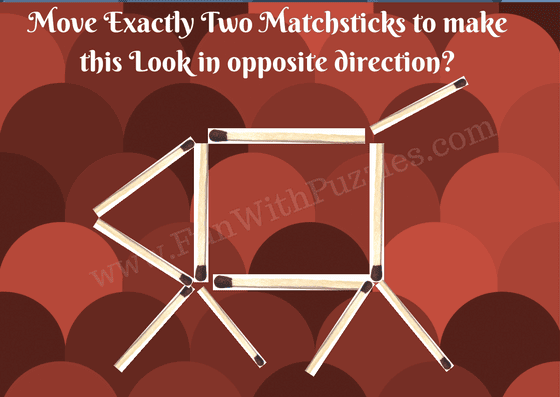Exciting matchstick puzzles for kids. Test their skills with visual challenges and find the answers to these captivating brain teasers.

As a kid, I used to play with matchstick puzzles. By taking a matchstick we used to make patterns and challenge friends to solve these puzzles. Earlier I published Matchstick Math Puzzles for Kids in which one has to move one matchstick to solve the given mathematical equation correctly. These puzzles were very much liked by the audience, then I created Matchstick Math Puzzles for Teens in which one has to move exactly two matchsticks to make the given Math equations correct. Today's matchstick puzzles are not mathematical. However, these are observational or visual puzzles.

## How to Solve Matchstick Picture Puzzle Questions

In each of these matchstick puzzles, one picture is given and one has to change the direction of this picture by moving a specific number of matchsticks to a new position. The number of matchsticks that will be required to be moved will differ from puzzle to puzzle and the specific count is mentioned in each of the puzzles. These are very easy puzzles. Kids will like it and find it challenging. However, teens and adults may find these puzzles easy. Let us see how many of these puzzles you can solve without looking at the answers?

The answers to these puzzles are given at the end of this post. Please post your answer in the comment section of this post.1. Matchstick Puzzle for kids-Donkey2. Matchstick Puzzle for kids-Fish3. Matchstick Puzzle for kids-Pattern4. Matchstick Puzzle for kids-Glass5. Matchstick Puzzle for kids-Dog

#### 1 comment:

Anya Linley said...

Nice selection. I have to disagree with how you've worded the first question though. The donkey doesn't face the opposite direction. Just a different diretion.